# pysampling¶https://github.com/anyoptimization/pysampling

# Installation¶

The framework is available at the PyPi Repository:

pip install -U pysampling


# Usage¶

The method to be used for sampling using different algorithm must be import from pysampling.sample. Here, we use Latin Hypercube Sampling to generate 50 points in 2 dimensions.

:

from pysampling.sample import sample

X = sample("lhs", 50, 2)


Then, we recommend using matpotlib or other visualization libraries to have a look at the results:

:

import matplotlib.pyplot as plt

plt.scatter(X[:, 0], X[:, 1], s=30, facecolors='none', edgecolors='r')
plt.show()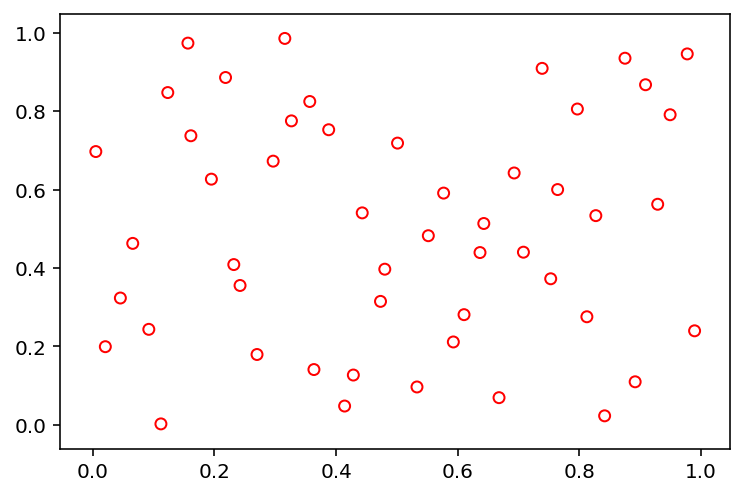# Features¶

So far our library provides the following implementations:

• Random (‘random’)

• Latin Hypercube Sampling (‘lhs’)

• Sobol (‘sobol’)

• Halton (‘halton’)

The initialization of each of those will be shown in the following. Let us first define a method that helps us to visualize them in a 2d space.

:

import matplotlib.pyplot as plt

def show(X):
plt.scatter(X[:, 0], X[:, 1], s=30, facecolors='none', edgecolors='r')
plt.show()


## Random (‘random’)¶

:

X = sample("random", 50, 2, seed=1)
show(X)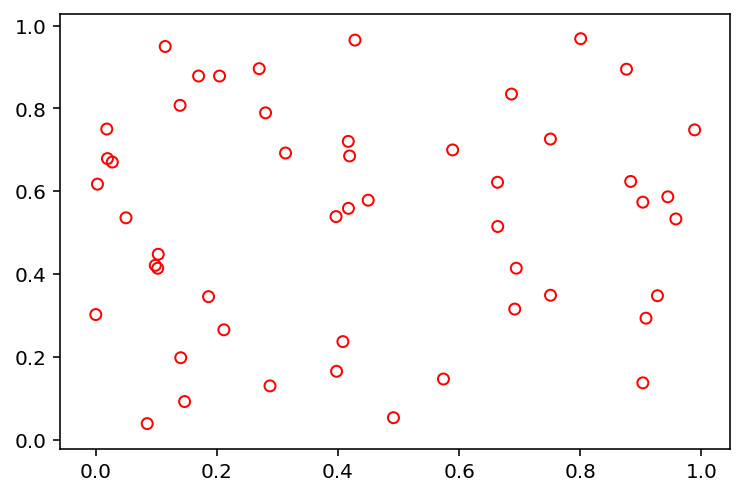## Latin Hypercube Sampling (‘lhs’)¶

:

X = sample("lhs", 50, 2, seed=1)
show(X)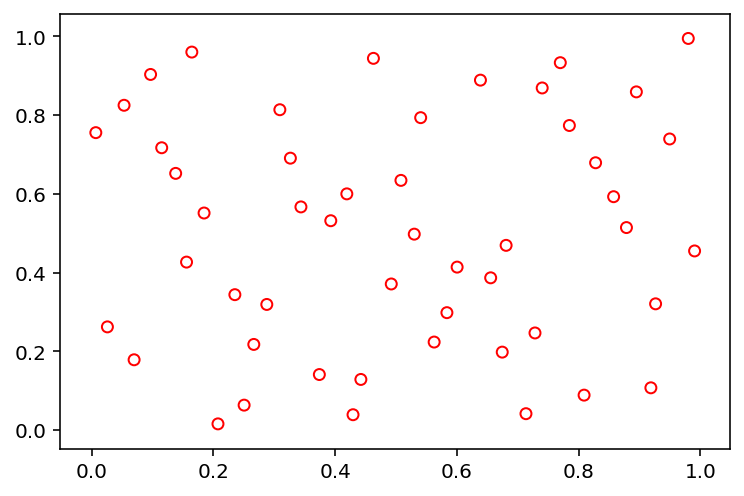## Sobol (‘sobol’)¶

:

X = sample("sobol", 84, 2)
show(X)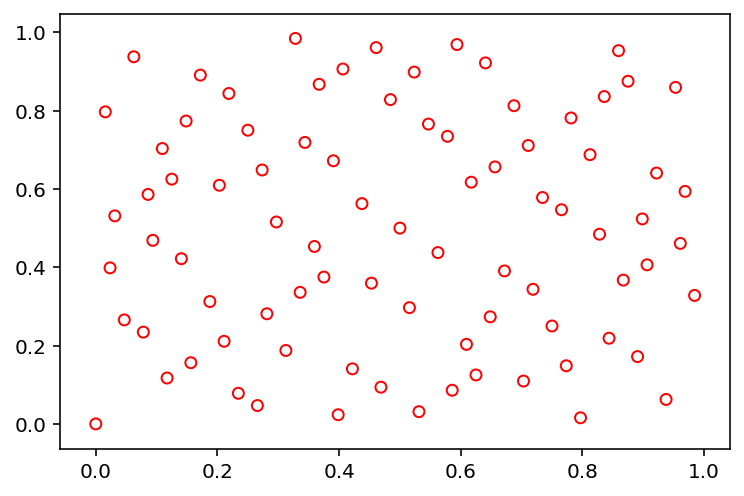:

X = sample("sobol", 84, 2, n_skip=100, n_leap=10)
show(X)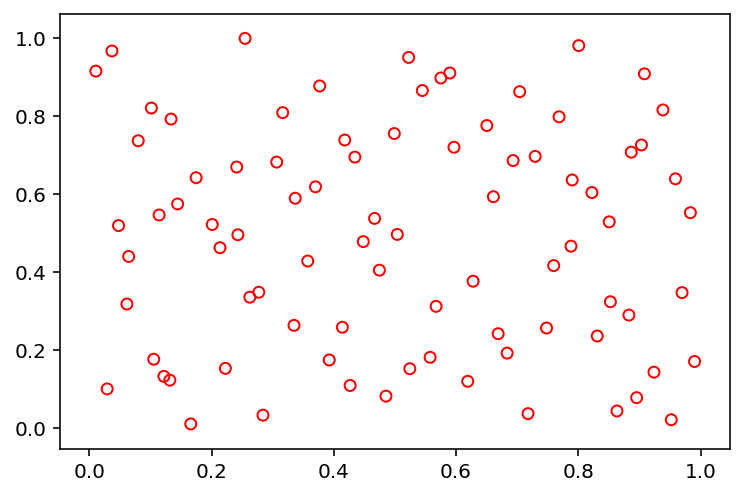## Halton (‘halton’)¶

:

X = sample("halton", 100, 2)
show(X)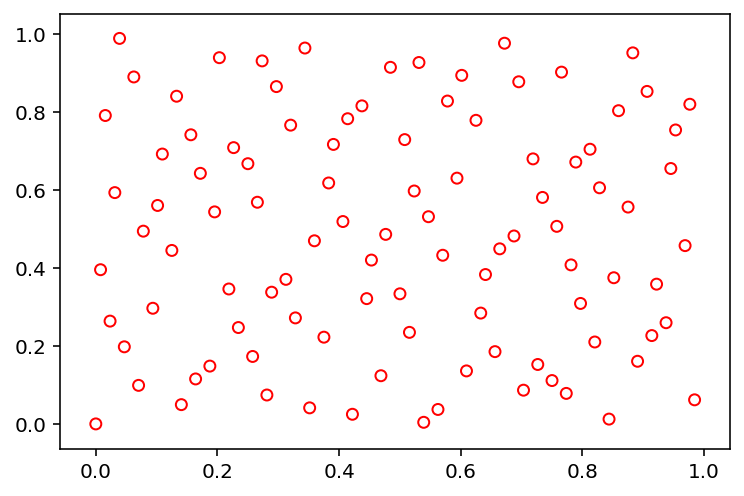# Contact¶

My personal homepage

Feel free to contact me if you have any question:

Julian Blank (blankjul [at] egr.msu.edu)
Michigan State University
Computational Optimization and Innovation Laboratory (COIN)
East Lansing, MI 48824, USA